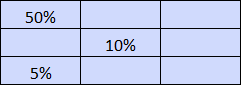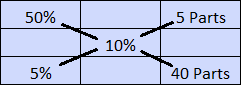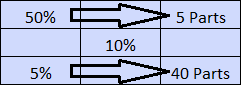# PTCB Math Quiz

Use this PTCB Math Quiz to practice your pharmacy math skills. Some of these questions require measurement conversions, so you may want to start with our measurement conversion quiz. These pharmacy tech practice math questions cover roman numerals, percentage calcuations, proportions, alligation, dosing calculations, and more. Detailed explanations are provided which show exactly how to solve these challenging problems. Get started on your test prep right now with our PTCB Math Quiz!

Congratulations - you have completed .

You scored %%SCORE%% out of %%TOTAL%%.

Your performance has been rated as %%RATING%%

 Question 1

### How is 34 written in roman numerals?

 A XXXIIII B XXLIV C XXXIL D XXXIV
Question 1 Explanation:
In the Roman numeral system:

$\text{I} = 1$, $\text{V} = 5$, $\text{X} = 10$, $\text{L} = 50$, $\text{C} = 100$

The ones, tens, fifties, and hundreds must be treated as separate items. It is not correct to use four identical symbols to represent a number. So a 4 would not be written as IIII, but instead IV, where it is treated as the difference of the two values:
IV = 5 − 1 = 4

30 = XXX and 4 = IV, so:

34 = XXXIV
 Question 2

### Convert this roman numeral to a number: LII

 A 52 B 12 C 102 D 110
Question 2 Explanation:
Be sure to know the Roman numeral system:

$\text{I} = 1$, $\text{V} = 5$, $\text{X} = 10$, $\text{L} = 50$, $\text{C} = 100$

L = 50 and II = 2, so:

LII = 52
 Question 3

### A 30 mL dose of nighttime cough suppressant contains 12.5 mg of doxylamine succinate. How many milligrams of doxylamine succinate are in the entire 354 mL bottle?

 A 240 B 128.32 C 375 D 147.5
Question 3 Explanation:
To solve this type of problem you should setup a proportion:

$\dfrac{12.5 \text{ mg}}{30 \text{ mL}} = \dfrac{x \text{ mg}}{354 \text{ mL}}$

Then you can cross multiply:
$30(x) = (12.5)(354)$
$30x = 4,425$

Divide both sides by $30$ to solve for $x$:
$x = 147.5$
 Question 4

### The pharmacy technician can reconstitute a 100 mL bottle of Amoxycillin in 50 seconds. How many of these bottles can the technician reconstitute in 30 minutes?

 A 32 B 36 C 34 D 38
Question 4 Explanation:
This is another proportion problem. Note that times are given in both seconds and minutes. You need to choose one or the other. We will use seconds, so the first step is to convert 30 minutes to seconds.

There are 60 seconds in a minute:

$\require{cancel} 30 \cancel{\text{minutes}} \cdot \frac{60 \text{ seconds}}{1 \cancel{\text{minute}}}$ $= 1800 \text{ seconds}$

Then setup the proportion:

$\frac{1 \text{ bottle}}{50 \text{ seconds}} = \frac{x \text{ bottles}}{1800 \text{ seconds}}$

Then you can cross multiply:
$50(x) = (1)(1800)$
$50x = 1800$
$x = 36$
 Question 5

### The pharmacy recieves a controlled substance order from their wholesaler. There are a total of 435 pills. Of this total, 17% are C2, 19% are C3, 20% are C4, and 44% are C5. How many C3 pills were in the order?

 A 74 B 87 C 19 D 83
Question 5 Explanation:
There are 435 pills, and 19% are C3. To find the number of C3 pills, multiply the total by 0.19:

$435 × 0.19 = 82.65$

This can be rounded to 83.
 Question 6

### Of all the prescriptions received at the pharmacy last Tuesday, 892 were refills. The remainder were new prescriptions, with 168 from patient drop-offs, 183 coming in electronically, and 69 coming in by phone. What percentage of the total prescriptions received were new drop-offs?

 A 14% B 52.5% C 12.8% D 16.8%
Question 6 Explanation:
First calculate the total prescriptions received:
$892 + 168 + 183 + 69$ $= 1,312$

Then take the number of new drop-off prescriptions (168) and divide it by the total number:
$168 ÷ 1,312 = 0.128$

To convert a decimal to a percentage, multiply by 100:
$0.128 × 100 = 12.8\%$
 Question 7

### A patient comes to the pharmacy counter with a prescription for Amoxicillin 250mg/5mL and the directions indicate 1¼ tsp po bid x 10d. What is the total quantity needed for dispensing?

 A 125 mL B 150 mL C 187.5 mL D 200 mL
Question 7 Explanation:
Recall that 1 teaspoon equals 5 mL. So 1¼ teaspoons equals 6.25 mL. Since it is twice a day (bid) for 10 days you must calculate:

$6.25 × 2 × 10 = 125 \text{ mL}$
 Question 8

### What volume of a drug for injection 100mg/45mL should be used for 250mg?

 A 45 mL B 100 mL C 112.5 mL D 250 mL
Question 8 Explanation:
To solve this type of problem you should setup a proportion:

$\dfrac{100 \text{ mg}}{45 \text{ mL}} = \dfrac{250 \text{ mg}}{x \text{ mL}}$

Then you can cross multiply:
$100(x) = (45)(250)$
$100x = 11,250$

Divide both sides by $100$ to solve for $x$:
$x = 112.5\text{ mL}$
 Question 9

### The pharmacist is preparing 200 mL of a famotidine suspension containing 75mg/5mL. How many 20 mg tablets of famotidine will be needed?

 A 3,000 B 40 C 150 D 400
Question 9 Explanation:
This is another proportion problem. First you must figure out how many milligrams of famotidine will be needed:

$\dfrac{75\text{ mg}}{5\text{ mL}} = \dfrac{x \text{ mg}}{200\text{ mL}}$

Cross multiply:
$5(x) = (75)(200)$
$5x = 15{,}000$

Divide both sides by $5$ to solve for $x$:
$x = 3{,}000$

Since there are 3,000 milligrams needed, you can divide this by 20 to find out how many 20 mg tablets are needed:
$3{,}000 ÷ 20 = 150$
 Question 10

### You need 800 mL of 10% dextrose solution. How much of your 50% and 5% dextrose solution will you need to mix?

 A Mix 89 mL of the 50% and 711 mL of the 5%. B Mix 122 mL of the 50% and 678 mL of the 5%. C Mix 111 mL of the 50% and 889 mL of the 5%. D Mix 100 mL of the 50% and 700 mL of the 5%.
Question 10 Explanation:
This is an alligation problem, so you should setjup a tic-tac-toe table. The highest concentration goes in the upper left corner and the lowest concentration in the lower left corner. The desired concentration goes in the middle:Subtract diagonally across the middle, making sure you subtract the smaller number from the larger number. Add the word 'parts' next to the result:Now the number of parts needed for each concentration is lined up across the table:So you need 5 parts of the 50% and 40 parts of the 5%, for a total of 45 parts.

Amount of 50% needed will be: $\dfrac{5}{45} × 800 = 89$

Amount of 5% needed will be $\dfrac{40}{45} × 800 = 711$
 Question 11

### How is 59 written in roman numerals?

 A CIX B LVIII C LIX D ILX
Question 11 Explanation:
Be sure to know the Roman numeral system:

$\text{I} = 1$, $\text{V} = 5$, $\text{X} = 10$, $\text{L} = 50$, $\text{C} = 100$

L = 50
IX = 9

59 = LIX
 Question 12

### Convert this roman numeral to a number: CXXVI

 A 76 B 116 C 91 D 126
Question 12 Explanation:
You should know your Roman numerals by now:

$\text{I} = 1$, $\text{V} = 5$, $\text{X} = 10$, $\text{L} = 50$, $\text{C} = 100$

C = 100
XX = 10 + 10 = 20
V = 5
I =1

CXXVI = 126
 Question 13

### Which of the following quantities will fill a 16 ounce container the most without causing any overflow?

 A 600 mL B 465 mL C 485 mL D 400 mL
Question 13 Explanation:
Recall that 1 oz = 30 mL. So 16 oz = 480 mL. Of these answers choices 485 mL is the closest to 480 mL, but that would cause overflow. The next lowest answer is correct, 465 mL.
 Question 14

### A bottle of fluticasone propionate nasal spray provides 120 metered sprays. Each spray delivers 50 mcg of fluticasone propionate in 100 mg of formulation through the nasal adapter. A prescription is filled for, “Three bottles, 2 sprays each nostril, qd.” How many days will this supply last?

 A 60 B 90 C 180 D 30
Question 14 Explanation:
Since the prescription states 2 sprays per nostril, and people have 2 nostrils, there will be 4 four sprays per day (qd is the abbreviation for daily). Each bottle has 120 sprays, so each bottle will last for:
$120 ÷ 4 = 30 \text{ days}$

Since there are 3 bottles:
$3 × 30 = 90 \text{ days}$
 Question 15

### A bottle with 236 milliliters of liquid allergy medication contains Diphenhydramine HCl 12.5mg/5mL. How milligrams of Diphenhydramine HCl are in the bottle?

 A 590 B 94.4 C 2,950 D 47.2
Question 15 Explanation:
Setup your proportion with $x$ representing the milligrams of Diphenhydramine HCl that are in the bottle:

$\dfrac{12.5 \text{ mg}}{5 \text{ mL}} = \dfrac{x \text{ mg}}{236 \text{ mL}}$

Cross multiply:
$(5)(x) = (12.5)(236)$
$5x = 2{,}950$

Divide both sides by $5$ to solve for $x$:
$x = 590$
 Question 16

### A bottle with 8 fluid ounces of liquid allergy medication contains 590 mg of Diphenhydramine HCl. How many grams of Diphenhydramine HCl are in 1 gallon of this medication?

 A 4.72 B 9,440 C 94.4 D 9.44
Question 16 Explanation:
There are two steps to solve this problem. Convert the milligrams in 8 fluid ounces to gallons, and then convert from milligrams to grams.

Recall that there are 8 fluid ounces in a cup. Which means there are 16 fluid ounces in a pint, 32 fluid ounces in a quart, and 128 fluid ounces in a gallon. How many cups are in a gallon?
$128 ÷ 8 = 16$

Multiply 590 mg by 16 to figure out how many mg are in 1 gallon:
$590 × 16 = 9{,}440 \text{ mg}$

The question asks for grams. There are 1,000 mg in 1 gram:
$9{,}440 ÷ 1{,}000$ $= 9.44 \text{ grams}$
 Question 17

### A child's prescription for ceftriaxone is 50mg/kg per day in equally divided doses every 12 hours. How many grams of the drug will be administered over the first 5 days if the child weighs 80 pounds?

 A 18 B 20,000 C 9 D 20
Question 17 Explanation:
The first step to solving this is to convert the child's weight to kilograms. Recall that 1 pound is equal to 0.454 kilograms:

$\require{cancel} 80 \cancel{\text{lb}} \cdot \dfrac{0.454 \text{ kg}}{1 \cancel{\text{lb}}}$ $= 36.32 \text{ kg}$

The child's weight can be rounded to 36 kg. Since the daily dose is 50mg/kg, the child's weight can be multiplied by 50:

$36 \cancel{\text{kg}} \cdot \dfrac{50 \text{ mg}}{\cancel{\text{kg}}}$ $= 1{,}800 \text{ mg (per day)}$

Over 5 days:
$5 × 1{,}800 = 9{,}000 \text{ mg}$

The final step is to convert this to grams:

$9{,}000 \cancel{\text{mg}} \cdot \dfrac{1 \text{ g}}{1{,}000 \cancel{\text{mg}}}$ $= 9 \text{ g}$
 Question 18

### The pharmacy technician knows that 600 mL of 20% dextrose solution contains _____ grams of dextrose.

 A 18 B 12 C 180 D 120
Question 18 Explanation:
To answer this type of problem you must know that percentage strength is conventionally given as weight to volume (w/v), where 20% means:

$\dfrac{20 \text{ g}}{100 \text{ mL}}$

The problem can be solved by setting up a proportion:

$\dfrac{x \text{ g}}{600 \text{ mL}} = \dfrac{20 \text{ g}}{100 \text{ mL}}$

$(100)(x) = (600)(20)$

$100x = 12{,}000$

$x = 120 \text{ g}$
Once you are finished, click the button below. Any items you have not completed will be marked incorrect.
There are 18 questions to complete.
 ← List →